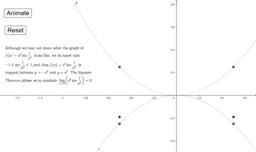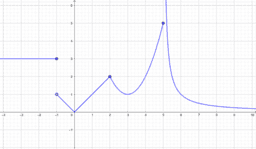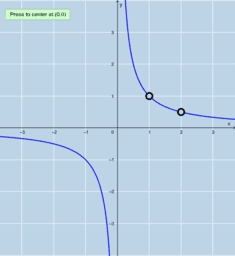# Theorems of Differential Calculus

Includes:
• The Squeeze Theorem
• The Intermediate Value Theorem
• The Extreme Value Theorem
• The Mean ValueTheorem
• L'Hopital's Rule
•### The Theorems of Differential Calculus

•### Mean Value Theorem: Quick Intuitive Tests

•### Hypotheses of the Theorems of Calc 1

•